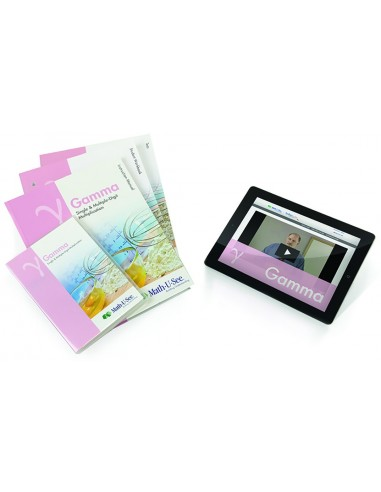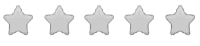See inside this book

# Math-U-See Gamma Level Up Set

\$88.00
No tax
Demme Learning
Math
3
P-MA03-61Leave a Review

#### Description

New! Math-U-See Gamma - Level Up Set

Math-U-See is a complete, skill-based, multi-sensory homeschool math curriculum for grades K-12 that?s designed to teach students skills in a logical sequence.

The Gamma Level Up Set is for customers who already have the required Integer Block Kit, this Set includes Gamma: Instruction Manual with complete solutions, Instruction DVD, Student Workbook, Tests booklet, and 12 months of access to our new Gamma Digital Pack, which includes the Skip Count Songs MP3s and Songbook PDF.

Once students have mastered the concepts of addition and subtraction (covered in Alpha and Beta), they are ready for multiplication. Gamma teaches single-digit facts and multiple-digit multiplication skills.

Major Concepts and Skills Include:

Using strategies based on place value and properties of operations to multiply Fluently multiplying any combination of whole numbers Solving for an unknown factor Solving abstract and real-world problems involving addition, subtraction, and multiplication Measuring and computing area Relating concepts of area to addition and multiplication

Skip counting as a precursor to multiplication Adding and subtracting time in hours and minutes Multiplying, adding, and subtracting U.S. currency and standard units of measure Representing, recording, and interpreting data Understanding of basic fractions Estimating and solving measurement problems